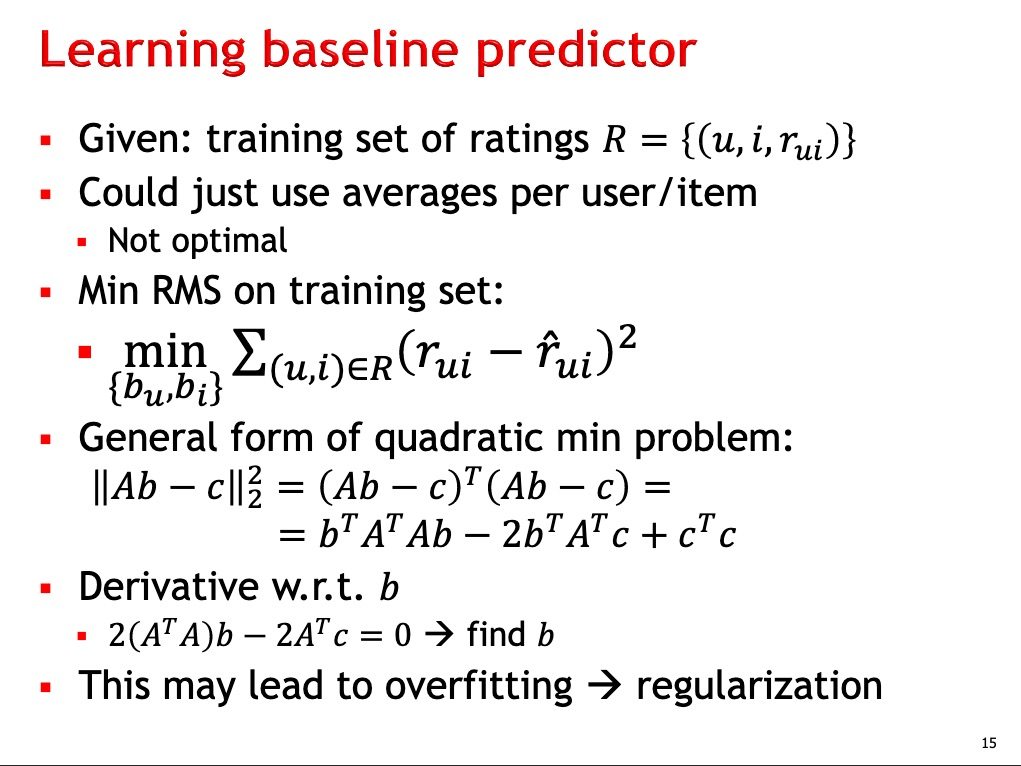### min RMS and quadratic form expression

Hello,
In the lecture 7 (slide 15), I'm stuck with understanding what's the meaning of matrix A and vector c in the sense of that problem.I don't really see the link between those dimensions (key notion in next slides) and this minimisation problem. I must be missing linear algebra principles, could you help me see better ?

Thanks a lot

Top comment

I would refer you to HW4 Ex2, where you need to find a way to write the RMS expression in matrix form as $$Ab-c$$, so as to apply the general formula for least squares. One useful hint is that $$A$$ will have one row for each pair $$(u,i)$$ where there is a rating in the training set.
I hope this helps!
Best,
MG

Page 1 of 1